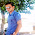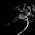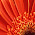VHDL coding tips and tricks: Fixed Point Operations in VHDL : Tutorial Series Part 2

## Sunday, March 28, 2010

### Fixed Point Operations in VHDL : Tutorial Series Part 2

This article is a continuation of the tutorial series on fixed_pkg library.In this article I will talk about, type conversions and assignments of signals of the fixed point data type.I assume that you have read Part 1 of the series.
Let us see how a signal assignment is made.Suppose I have a signal of unsigned fixed point type.I can assign a value (say 9.75 ) in the following way:

signal n1 : ufixed(4 downto -3);
n1 <= to_ufixed (9.75,4,-3);   -- n1 ="01001110"

Here TO_UFIXED is the conversion function used to convert the value "9.75" to binary number in the prescribed range.For a signed number you can use the function "TO_SFIXED".

--an example for TO_SFIXED.
signal n2 : sfixed(4 downto -3);
n1 <= to_sfixed (-9.75,4,-3);  -- n2 = "10110010"

Assignments can be made in a different way also.This is done by using a signal name as a parameter, instead of actual range values.

--Both n1 and n3 contains the same value.
signal n1,n2,n3 : ufixed(4 downto -3);
n1 <= to_ufixed (9.75,n1);   -- n1 ="01001110"
n3 <= to_ufixed (9.75,n2);   -- n3 ="01001110"
Another method of type conversion is by using signal_name'high and signal_name'low attributes.See the below example.
--Type conversion using attributes.
signal n1,n2,n3 : ufixed(4 downto -3);
n1 <= to_ufixed (9.75,n1'high,n1'low);   -- n1 ="01001110"
n3 <= to_ufixed (9.75,n2'high,n2'low);   -- n3 ="01001110"

All the above methods applies equally to TO_SFIXED conversion function also.
Another useful function available in fixed_pkg is resize().This function is used to fix the size of the output.But while changing the size of the output the signal value may get rounded off or get saturated.

--An example for resize() function.
signal n1 : ufixed(4 downto -3);
signal n2 : ufixed(5 downto -5);
n1 <=  to_ufixed (9.75,n2);   -- n1 = "01001110"
n2 <= resize(n1,n2);          -- n2 ="00100111000"

--Resizing functions can be called in the following ways also:
--Method 1
n2 <= resize(n1,n2'high,n2'low);
--Method 2
n2 <= resize(n1,5,-5);

Take the following statements.
n2 <=  to_ufixed (31.75,n2);      -- n2 ="01111111000"
n1 <= resize(n2,n1);              -- n1 = "11111110"
In the above code snippet the values are assigned without any rounding off because the value "31.75" matched with the range of 'n1'.
Now take the next two statements:
n2 <=  to_ufixed (32.75,n2);      -- n2 ="10000011000"
n1 <= resize(n2,n1);              -- n1 = "11111111"
Here the range of 'n1' is not sufficient for the value "32.75",so while resizing it we got all 1's in the output.While writing your code, you should take care of such things.

Note :- Beware of the following type of assignments:

signal n1 : ufixed(4 downto -3);
signal n2 : ufixed(3 downto -4);
n1 <=  to_ufixed (5.75,n1);      -- n1 = "00101110" = 5.75
n4 <= n1;                                -- n4 = "00101110" = 2.875

Here the compiler will not show any error or warning on 4th line because the size and type of both the signals are same.But as you can see this is not what we expected.Take care while writing your code using fixed_pkg.You may get unexpected results even if you are careless about a single line of code.

#### 8 comments:

1.This tutorial was inspired from fixed_pkg" documentation by David Bishop.The original document can be downloaded from
http://www.vhdl.org/fphdl/Fixed_ug.pdf

1.2.Does the line [n1 <= to_sfixed (-9.75,4,-3); -- n2 = "10110010"] have a typo ? Should it be [n2 <= to_sfixed (-9.75,4,-3); -- n2 = "10110010"] ?

Thanks for the tutorial.

3.Does the line [n4<=n1;-- n4 = "00101110" = 2.875] have a typo ? Where is n4 defined ? Should it be n2 ?

4.@Jim: thanks for noticing the typos.

5.I am getting confused in s_fixed and u_fixed .

signal n1 : ufixed(4 downto -3);
n1 <= to_ufixed (9.75,4,-3); -- n1 ="01001110"

signal n2 : sfixed(4 downto -3);
n1 <= to_sfixed (-9.75,4,-3); -- n2 = "10110010"

Why is there a difference in the binary values for sfixed and ufixed ? I understand the format of ufixed (01001 ==>9 and (110 =>0.75) but what is the format for s_fixed ?

1.one is signed representation which means its two's complementary, and another is unsigned format.

6.how to add library ieee_proposed